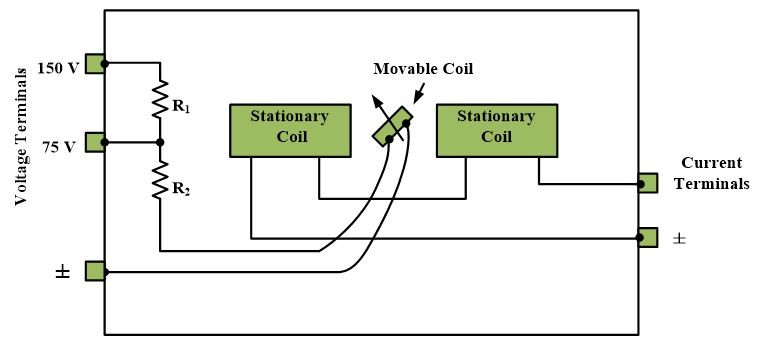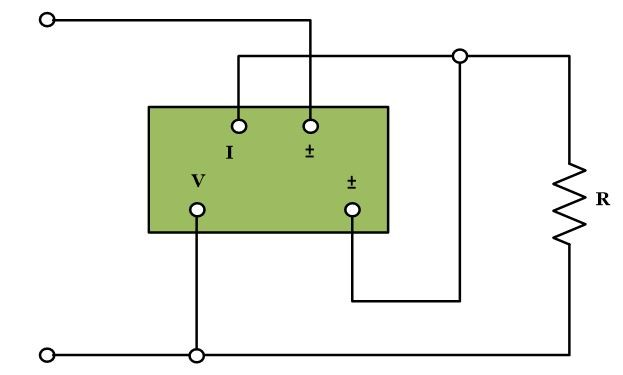Home / Instrumentation / Power Measurement In a Three-Phase System using Wattmeter

Power Measurement In a Three-Phase System using Wattmeter

Want create site? Find Free WordPress Themes and plugins.

Wattmeter is an instrument, used to measure power in a circuit using current and voltage of the circuit.

The wattmeter is essentially an electrodynamic mechanism, in which the stationary and movable coils separately measure the current and voltage of the circuit, as shown in Fig.The stationary coil is designed to handle the current of the circuit, whereas the moving coil is connected in series with a multiplying resistor and designed to respond to the voltage of the circuit. As the torque of the moving coil depends directly on the magnetic fields produced by both coil, the moving coil deflection is proportional to the product of voltage and current. Hence the instrument is calibrated directly in watts.

In an AC circuit, the voltage and current are generally not in phase so that their product does not equal the power. Rather, the power equals the product of voltage and current, the apparent power, and a term called the power factor. Often one does not know the Power factor of an AC circuit and cannot determine the power from separate voltage and current measurements. Yet, the electrodynamic wattmeter allows one to determine directly the power in an AC circuit.

Wattmeters have one voltage and one current terminal signed with a ± symbol. For an upscale deflection, current must simultaneously enter both signed terminals or leave both signed terminals. A correct wattmeter connection is shown in the following figure.The wattmeter connection shown in above figure is a short shunt connection since the voltage element of the wattmeter is across the load only. The wattmeter reads not only the load power but also the power lost in a voltage element. Knowing the resistance of the appropriate element, one can correct for the instrument power loss.

Example

The wattmeter in above figure reads 200 W when the line voltage is 150 V. if the resistance of the voltage element is 4 kW, what is true load power?

Solution

$P=reading-meter~loss=200-\frac{{{V}^{2}}}{R}$

$=200-\frac{{{150}^{2}}}{4*{{10}^{3}}}=200-5.6=194.4~W$

Both the voltage and current elements of a wattmeter have ratings and these ratings should not be exceeded. However, it is possible to exceed either the voltage or current rating even though the wattmeter pointer does not go off the scale. Therefore, one should have an idea of voltage and current being applied to a wattmeter. A shunt can be used so as not to exceed a current rating and a series resistor can be used so as not to exceed a voltage rating.

Did you find apk for android? You can find new Free Android Games and apps.